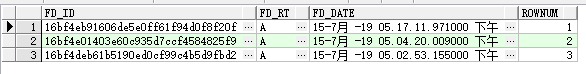http://www.360doc.com/showweb/0/0/860281883.aspx

`SELECT * FROM tableName where fd_rt = 'A' --and rownum=1 ORDER BY fd_date DESC`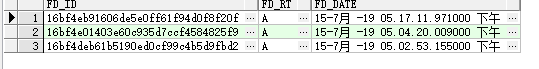SELECT * FROM tableName where fd_rt = 'A'

and rownum=1

ORDER BY fd_date DESC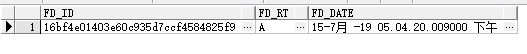`SELECT t.* from(SELECT * FROM tableName where fd_rt = 'A' ORDER BY fd_date DESC) t WHERE rownum = 1`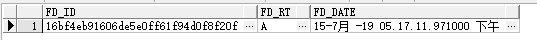rownum作为伪列实际上查询结果为：

`SELECT tableName .*,rownum FROM tableName where fd_rt = 'A' --and rownum=1 ORDER BY fd_date DESC`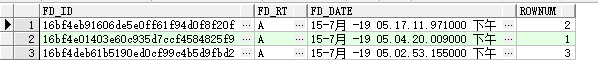`SELECT t.*,rownum from(	SELECT * FROM tableName where fd_rt = 'A' ORDER BY fd_date DESC) t`